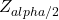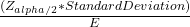Question

A university dean of students wishes to estimate the average number of hours students spend doing homework per week. The standard deviation from a previous study is 6.2 hours. How large a sample must be selected if he wants to be 99% confident of finding whether the true mean differs from the sample mean by 1.5 hours

1.RobertKer

The Sample Size Need To be at least 114

Step-by-step explanation:

According to the Question,

• Given, A university dean of students wishes to estimate the average number of hours students spend doing homework per week. The standard deviation from a previous study is 6.2 hours.

The Maximum Error(E) is given as 1.5 hours and the standard deviation(σ) is 6.2 hours.

• Now, For 99% Confident the level of value α is 0.01.

α/2 = 0.01/2 ⇒ 0.005

• Thus, From The table, We get that the value ofis 2.58.

Hence, the sample size n can be found as

n = {n = {2.58 × 6.2}² / 1.5²

n = 113.72 .

So, The Sample Size Need To be at least 114.